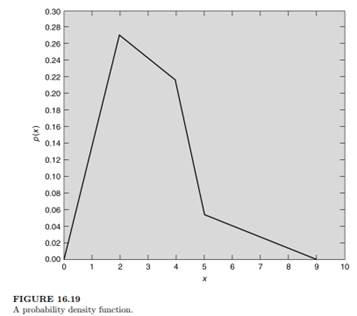### Create an Account

Home / Questions / Using the graph of the probability density function of an underlying population presented ...

# Using the graph of the probability density function of an underlying population presented in Figure 519 determine a The percent probability that one randomly selected value from this population w

Using the graph of the probability density function of an underlying population presented in Figure 5.19, determine

(a) The percent probability that one randomly selected value from this population will be between the values of two and five. If a sample of 20 values are drawn randomly from this population, determine

(b) How many will have values greater than two and

(c) How many will have values greater than five.Jul 26 2020 View more View LessSubscribe To Get Solution